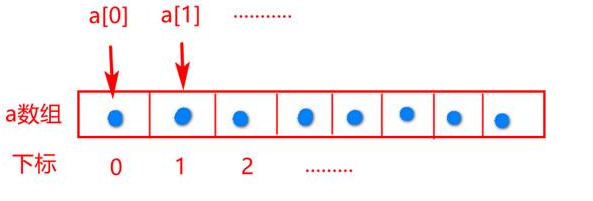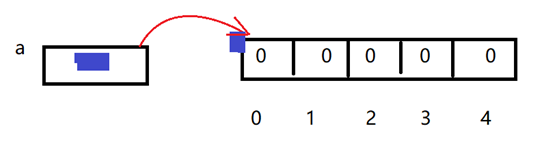## JAVA基础(变量+方法+数组)

2020/8/11 19:50:18 文章标签:

# 变量

``````public class Test {
//1、成员变量：在类里方法外是
//作用范围就是整个类里
//可以不初始化，也会有默认值
int age = 20;
int sum = 30;
public static void main(String[] args) {
//2局部变量：在方法里是
//作用范围就是方法里，出了这个方法就不认识了
//必须初始化
int sum = 10;
System.out.println(sum);//10
//3、变量的就近原则，附近有同名的会去执行最近的
System.out.println(sum);//10
}
}
``````

# 方法

}

## 方法的重载

``````public class Test{
public static void main(String[] args) {

int a=10;
int b=20;
String name="lisi";
c1();
c1(a);
c1(a,b);
c1(a,b,name);
}
private static void f1(int a, int b, String name) {
System.out.println(a+b+name);//30name
}
private static void f1() {
System.out.println("f1()");
}
private static void f1(int a) {
System.out.println(a);
}
private static void f1(int a, int b) {
System.out.println(a+b);
}
}
``````

## 数组### 创建数组

int[] a = new int;int[] a ={1,2,3,4,5,6,7,8};

int[] a =new int[]{1,2,3,4,5};

``````将数组中存入hello
public class Test{
public static void main(String[] args) {
b1();
}
private static void b1() {
int[] i = new int[]{1,2,3,4,5};
System.out.println(Arrays.toString(i));
char[] a = new char;
a='h';
a='e';
a='l';
a='l';
a='o';
System.out.println(Arrays.toString(a));
}
}
``````

length属性获取数组长度

### 数组的遍历

for(int i=0;i<a.length;i++){
syso(a[i]);
}

``````遍历数组，存入1到10
public class Test7 {
public static void main(String[] args) {
a1();
}
private static void a1() {
int[] arr=new int;
for (int i = 0; i < arr.length; i++) {
arr[i]=i+1;
}
System.out.println(Arrays.toString(arr));
}
}
``````

### 数组工具类Arrays

Arrays.toString(数组)

Arrays.sort(数组)

Arrays.copyOf(数组，新的长度)

``````int[] a = Arrays.copyOf(arr, 10);//数组的复制，大于原来长度相当于扩容
System.out.println(Arrays.toString(a));//[12, 30, 20, 90, 34, 0, 0, 0, 0, 0]
System.out.println(a.length);//10
int[] a2 = Arrays.copyOf(arr, 3);//数组的复制，晓宇原来长度相当于截取前几个数据
System.out.println(Arrays.toString(a2));//[12, 30, 20]
System.out.println(a2.length);//10
``````

暂无相关的数据...